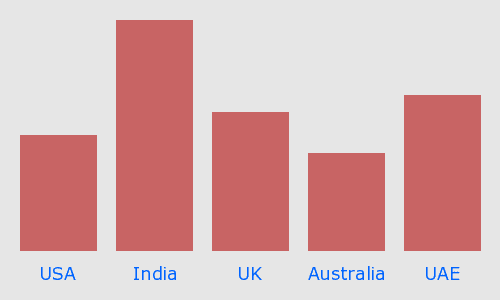## Thursday, August 18, 2011

### Create Dynamic Bar Chart in PHP

Using PHP GD Library Functions. we can create Bar chart in php with dynamic data,
here is my SimpleBar Chart class to create a dynamic bar chart for your projects. you can easily add any number of columns and values also you can change your color scheme on base class.Sample Bar Chart Using SimpleBar.class.php

SimpleBar.class.php

```<?php

class SimpleBar {
private \$xgutter = 20; // left/right margin
private \$ygutter = 20; // top/bottom margin
private \$bottomspace = 30; // gap at the bottom
private \$internalgap = 20; // space between bars
private \$cells = array(); // labels/amounts for bar chart
private \$totalwidth; // width of the image
private \$totalheight; // height of the image
private \$font; // the font to use

function __construct( \$width, \$height, \$font ) {
\$this->totalwidth = \$width;
\$this->totalheight = \$height;
\$this->font = \$font;
}

function addBar( \$label, \$amount ) {
\$this->cells[ \$label ] = \$amount;
}

private function _getTextSize( \$cellwidth ) {
\$textsize = (int)(\$this->bottomspace);
if ( \$cellwidth < 10 ) {
\$cellwidth = 10;
}
foreach ( \$this->cells as \$key=>\$val ) {
while ( true ) {
\$box = ImageTTFbBox( \$textsize, 0, \$this->font, \$key );
\$textwidth = abs( \$box );
if ( \$textwidth < \$cellwidth ) {
break;
}
\$textsize--;
}
}
return \$textsize;
}

function draw() {
\$image = imagecreate( \$this->totalwidth, \$this->totalheight );
\$red = ImageColorAllocate(\$image, 230, 230, 230);
\$blue = ImageColorAllocate(\$image, 200, 100, 100 );
\$black = ImageColorAllocate(\$image, 0, 100, 255 );

\$max = max( \$this->cells );
\$total = count( \$this->cells );
\$graphCanX = ( \$this->totalwidth - \$this->xgutter*2 );
\$graphCanY = ( \$this->totalheight - \$this->ygutter*2
- \$this->bottomspace );
\$posX = \$this->xgutter;
\$posY = \$this->totalheight - \$this->ygutter - \$this->bottomspace;
\$cellwidth = (int)(( \$graphCanX -
( \$this->internalgap * ( \$total-1 ) )) / \$total) ;
\$textsize = \$this->_getTextSize( \$cellwidth );

foreach ( \$this->cells as \$key=>\$val ) {
\$cellheight = (int)((\$val/\$max) * \$graphCanY);
\$center = (int)(\$posX+(\$cellwidth/2));
imagefilledrectangle( \$image, \$posX, (\$posY-\$cellheight),
(\$posX+\$cellwidth), \$posY, \$blue );
\$box = imageTTFbBox( \$textsize, 0, \$this->font, \$key );
\$tw = \$box;
ImageTTFText( \$image, \$textsize, 0, (\$center-(\$tw/2)),
(\$this->totalheight-\$this->ygutter), \$black,
\$this->font, \$key );
\$posX += ( \$cellwidth + \$this->internalgap);
}

imagepng( \$image );
}
}

?> ```

Example Bar Chart
```<?php
include("SimpleBar.class.php");

\$graph = new SimpleBar( 500, 300, "verdana.ttf" );
\$graph->draw();
?>

```View Demo    Download Script

1.2.